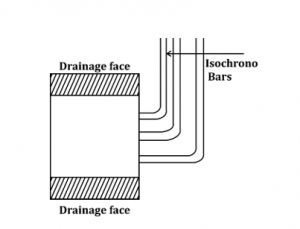Engineering Jobs   »   CIVIL ENGINEERING QUIZ

# GATE’22 CE:- Daily Practice Quiz 23-July-2021

Quiz: Civil Engineering
Exam: GATE
Topic: MISCELLANEOUS

Each question carries 2 mark
Negative marking: 1/3 mark
Time: 12 Minutes

Q1. A rigid bar is supported by three rods in the same vertical plane and equidistant. The outer rods are of brass and of length 600 mm and diameter 300mm. the central rod is of steel of 900 mm length and of 37.5 mm diameter. Calculate the force in the bars due to an applied force P, of bar remains horizontal after the load has been applied, Take E_S/E_b =2
(a) 0.44P, 0.66P
(b) 0.245P, 0.51P
(c) 0.212P, 0.55P

(d) 0.60P, 0.40P

Q2. The slope of isochrones at any point at a given time indicates the rate of change of
(a) effective stress with time
(b) effective stress with depth
(c) pore water pressure with depth
(d) pore water pressure with time

Q3. A fall in a canal bed is generally provided, if
(a) Ground slope exceeds the designed bed slope.
(b) Designed bed slope exceeds the ground slope.
(c) Ground slope is practically the same as the designed bed slope.
(d) Canal bed is flat.

Q4. For a road with caber of 3% and the design speed of 90km/hr, the minimum radius of the curve beyond which no superelevation is needed is
(a) 1680 m
(b) 948 m
(c) 406 m
(d) 1200 m

Q5. Fineness modulus of fine aggregate is 278 and coarse aggregate is 7.82 and the desired fineness modulus of mixed aggregate is 6.14. what is the amount of fine aggregate to be mixed with one part of coarse aggregate?
(a) 55%
(b) 50%
(c) 45%
(d) 40%

S1. Ans.(b)
Sol. Using 1 for Brass & 2 for steel
A_1=π/4×30^2=225π mm^2
A_2=π/4×〖37.5〗^2=351.56 π mm^2
From statical equilibrium equation,
2P_1+P_2=P—-(1)
From compatibility equation
δl_1=δl_2
(P_1 L_1)/(A_1 E_1 )=(P_2 L_2)/(A_2 E_2 )
P_2 =(P_1 A_2 E_2 L_1)/(A_1 E_1 L_2 )=(P_1×351.56π×2×600)/(225π×1×900)
P_2=2.08P_1
Substituting in (1).
2P_1+2.08P_1=P
▭(P_1=0.245P)
P_2 = 2.08 × 0.245P
▭(P_2=0.51P)
S2. Ans.(c)
Sol. the slope of isochromes indicates the rate of change of pore water pressure with depth of the soilmass pore water pressure will reduce with respect to time.S3. Ans.(a)
Sol. if ground slope exceeds the designed bed slope.
S4. Ans.(d)
Sol. Taking camber as superelevation,
e=3/100=0.03
0.03=V^2/225R=90^2/225R
R = 1200 m
S5. Ans.(b)
Sol. Let x fraction part of FA is to be mixed with 1 part of CA to form the mix aggregate.
278x + 7.82 = (1+x) 6.14
X = 0.5 (50%)

Sharing is caring!